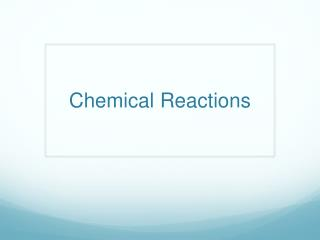DownloadDownload PresentationChemical Reactions

# Chemical Reactions

Download Presentation## Chemical Reactions

- - - - - - - - - - - - - - - - - - - - - - - - - - - E N D - - - - - - - - - - - - - - - - - - - - - - - - - - -
##### Presentation Transcript

1. Chemical Reactions

2. - Observing Chemical Change Properties and Changes in Matter • Matter can undergo both physical change and chemical change.

3. - Observing Chemical Change Asking Questions Properties and Changes of Matter Question Answer What are physical properties of matter? Physical properties are characteristics that can be observed without changing one substance into another. What is the evidence for chemical reactions? The evidence for chemical reactions is the formation of new substances and changes in energy.

4. Chemical Reactions • Rearrange atoms • Involve changes in energy • Exothermic: release energy (heat, fire, fizz, etc) • Endothermic: absorb energy (drop in temp.)

5. Evidence of Chemical Changes Cut along dotted lines and paste into your science notebook.

6. - Observing Chemical Change Properties and Changes in Matter • Chemical changes occur when bonds break and new bonds form.

7. - Describing Chemical Reactions Describing Chemical Reactions • Cellular phone messages make use of symbols and abbreviations to express ideas in shorter form. Similarly, chemists often use chemical equations in place of words.

8. - Describing Chemical Reactions What Are Chemical Equations? • Chemical equations use chemical formulas and other symbols instead of words to summarize a reaction. The arrow symbol is read YIELDS which means “results in” or “produces”

9. ___ CH4 + ___ O2 ___CO2 + ___ H2O Chemical Equations methane and oxygen yield carbon dioxide and water Reactants Products The molecules that combine are called REACTANTS and the molecules that result are called PRODUCTS

10. - Describing Chemical Reactions Chemical Formulas • The formula of a compound identifies the elements in the compound and the ratios in which their atoms are present.

11. - Describing Chemical Reactions Conservation of Mass • The principle of conservation of mass states that in a chemical reaction, the total mass of the reactants must equal the total mass of the products.

12. - Describing Chemical Reactions Balancing Chemical Equations • To describe a reaction accurately, a chemical equation must show the same number of each type of atom on both sides of the equation.

13. - Describing Chemical Reactions Balancing Chemical Equations • Magnesium metal (Mg) reacts with oxygen gas (O2), forming magnesium oxide (MgO). To write a balanced equation for this reaction, first write the equation using the formulas of the reactants and products, then count the number of atoms of each element.

14. Balancing Chemical Equations: Balance the equation for the reaction of sodium metal (Na) with oxygen gas (O2), forming sodium oxide (Na2O). - Describing Chemical Reactions Balancing Chemical Equations

15. Balancing Chemical Equations: Balance the equation for the reaction of tin (Sn) with chlorine gas (Cl2), forming tin chloride (SnCl2). - Describing Chemical Reactions Balancing Chemical Equations

16. - Describing Chemical Reactions Classifying Chemical Reactions • Many chemical reactions can be classified in one of three categories: synthesis, decomposition, or replacement.

17. Chemical Reactions Two or more elements or compounds combine to make a more complex substance A + B → AB AB → A + B Compounds break down into simpler substances Occurs when one element replaces another one in a compound AB + C → AC + B AB + CD → AC + BD Occurs when different atoms in two different compounds trade places A = Red B = Blue C = Green D = Yellow

18. Identifying Chemical Reactions Use the code below to classify each reaction. S = Synthesis D = Decomposition SR = Single Replacement DR = Double Replacement ____ P + O2 → P4O10 ____ Mg + O2 → MgO ____ HgO → Hg + O2 ____ Al2O3 → Al + O2 ____ Cl2 + NaBr → NaCl + Br2 ____ CuCl2 + H2S → CuS + HCl

19. Key Terms: Key Terms: Examples: Examples: closed system replacement coefficient synthesis decomposition - Describing Chemical Reactions Building Vocabulary Key Terms: Examples: chemical equation A chemical equation is a short, easy way to show a chemical reaction. In a closed system, matter is not allowed to enter or leave. Replacement is the process in which one element replaces another in a compound or two elements in different compounds trade places. reactant A substance you have at the beginning of a reaction is a reactant. A coefficient is a number in a chemical equation telling you how many atoms or molecules of a reactant or product take part in the reaction. product A new substance produced in a reaction is a product. Synthesis means combining two or more elements or compounds to make a more complex substance. conservation of mass Conservation of mass means that during a chemical reaction, matter is not created or destroyed. open system In an open system, matter can enter from or escape to the surroundings. Decomposition means breaking down compounds into simpler products.

20. End of Section:Describing Chemical Reactions